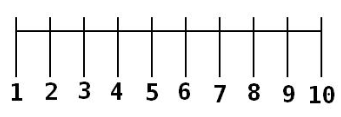# Number Line Math

Computational Thinker### Overview​

Number Line by the Math Learning Center is a useful tool for students to provide strategies for addition, subtraction, multiplication, and division. This extension also allows for creating number lines with fractions and negative numbers.

This activity card can be used in grades two to five and can be adjusted for the students the teacher is working with.

Students will:

• Be able to use a number line to assist them in math strategies.
• Be able to create an equation and also a written sentence.

Vocabulary Words:

1. Number line: A number line is a line on which numbers are marked at intervals, used to illustrate simple numerical operations.

2. Interval: An interval is a space between two things; a gap.

3. Equation: An equation is a statement that the values of two mathematical expressions are equal.

4. Toolbar: A toolbar is a strip of icons used to perform certain functions.

5. Drawing Tool: The drawing tool is a tool designated for drawing.

6. Pen Tool: The pen tool is a tool used for writing in a program.

To prepare for this lesson:

• The teacher should have students log onto the chrome web store and locate the extension Number Line by the Math Learning Center and add it to their Chromebook. They can also use the online version

• The teacher should have students add the app Number Line from the apple app store and add it to their iPad.

• Prior to lesson introduce the tools on the Number Line.

• Watch the two tutorial videos.

• See the Accommodations Page and Charts on the 21things4students website in the Teacher Resources.
• A mouse may be more helpful with younger students or those with dexterity issues for easier manipulation of the tools.

Directions for this activity:

1. The teacher provides student with a math equation to solve.

2. The teacher introduces either the online, extension, or app version of Math Center's Number Line app.

3. The students create or select the appropriate number line to solve the equation.

4. The student types the math equation as it was solved on the number line with the equation tool.

5. The student uses the pen tool to write a written math sentence.

Different options for assessing the students:

• Observations
• Check for understanding
• Have students write a number line equation for their ticket out the door.
• The students share their equations with the class and explain them.

MITECS: Michigan adopted the "ISTE Standards for Students" called MITECS (Michigan Integrated Technology Competencies for Students) in 2018.

Computational Thinker
5a. Students formulate problem definitions suited for technology-assisted methods such as data analysis, abstract models and algorithmic thinking in exploring and finding solutions.
5b. Students collect data or identify relevant data sets, use digital tools to analyze them, and represent data in various ways to facilitate problem-solving and decision-making.
5c. Students break problems into component parts, extract key information, and develop descriptive models to understand complex systems or facilitate problem-solving.
5d. Students understand how automation works and use algorithmic thinking to develop a sequence of steps to create and test automated solutions.

Browser: Chrome, Safari, Firefox, Edge, ALL

Apps and Extensions:
Number line app iOS
Number line extension in the Chrome Store

Websites:
Number Line from the Math Center

Number Line App Video Tutorial One

Number Line App Video Tutorial Two

##### CONTENT AREA RESOURCES

Explain how you solved the math equation on the number line.

Draw a picture of a number line and your mathematical explanation.

Use the number line solve equations.

CREDITS
This task card was created by Julie Hoehing, Lake Shore Public Schools, May 2018. Updated October 2023.